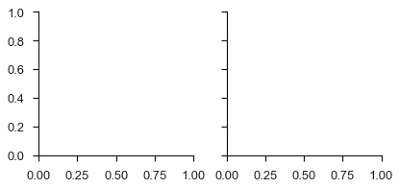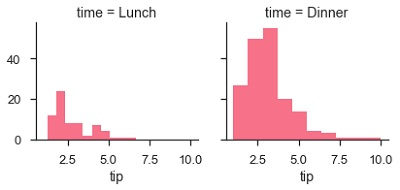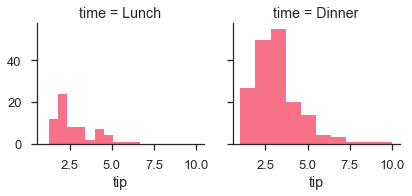# Seaborn - Facet Grid

#### Data Visualization using MatPlotLib & Seaborn

11 Lectures 4 hours

#### Seaborn with Python

11 Lectures 2.5 hours

#### Data Visualization in Python Using Seaborn Library

20 Lectures 2 hours

A useful approach to explore medium-dimensional data, is by drawing multiple instances of the same plot on different subsets of your dataset.

This technique is commonly called as “lattice”, or “trellis” plotting, and it is related to the idea of “small multiples”.

To use these features, your data has to be in a Pandas DataFrame.

## Plotting Small Multiples of Data Subsets

In the previous chapter, we have seen the FacetGrid example where FacetGrid class helps in visualizing distribution of one variable as well as the relationship between multiple variables separately within subsets of your dataset using multiple panels.

A FacetGrid can be drawn with up to three dimensions − row, col, and hue. The first two have obvious correspondence with the resulting array of axes; think of the hue variable as a third dimension along a depth axis, where different levels are plotted with different colors.

FacetGrid object takes a dataframe as input and the names of the variables that will form the row, column, or hue dimensions of the grid.

The variables should be categorical and the data at each level of the variable will be used for a facet along that axis.

### Example

```import pandas as pd
import seaborn as sb
from matplotlib import pyplot as plt
g = sb.FacetGrid(df, col = "time")
plt.show()
```

### OutputIn the above example, we have just initialized the facetgrid object which doesn’t draw anything on them.

The main approach for visualizing data on this grid is with the FacetGrid.map() method. Let us look at the distribution of tips in each of these subsets, using a histogram.

### Example

```import pandas as pd
import seaborn as sb
from matplotlib import pyplot as plt
g = sb.FacetGrid(df, col = "time")
g.map(plt.hist, "tip")
plt.show()
```

### OutputThe number of plots is more than one because of the parameter col. We discussed about col parameter in our previous chapters.

To make a relational plot, pass the multiple variable names.

### Example

```import pandas as pd
import seaborn as sb
from matplotlib import pyplot as plt